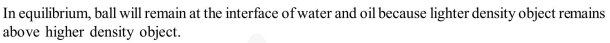Deepak Scored 45->99%ile with Bounce Back Crack Course. You can do it too!

# A ball is made of a material

Question:

A ball is made of a material of density $\rho$ where $\rho_{\text {oil }}<\rho<\rho_{\text {water }}$ with $\rho_{\text {oil }}$ and $\rho_{\text {water }}$ representing the densities of oil and water, respectively. The oil and water are immiscible. If the above ball is in equilibrium in a mixture of this oil and water, which of the following pictures represents its equilibrium position ?

1.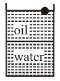2.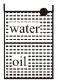3.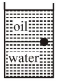4.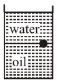Correct Option: , 3

Solution: